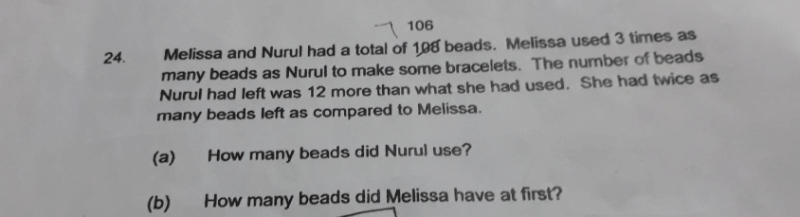# Questiona) All the information in the question can be represented in table form below:

 Used Left Total Melissa 3 units 0.5 unit + 6 3.5 units + 6 Nuru 1 unit 1 unit +12 2 units +12

3.5 units + 6 + 2 units + 12 = 106
5.5 units +18 = 106
5.5 units = 88
1 unit = 88 ÷ 5.5

b) 3.5 units → 16 x 3.5
= 56
56 + 6 = 62 beads

Check:

 Used Left Total Melissa 16 x 3 = 48 8 + 6 = 14 62 Nuru 16 x 1 = 16 16 + 12 = 28 44